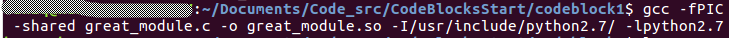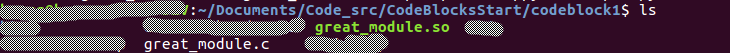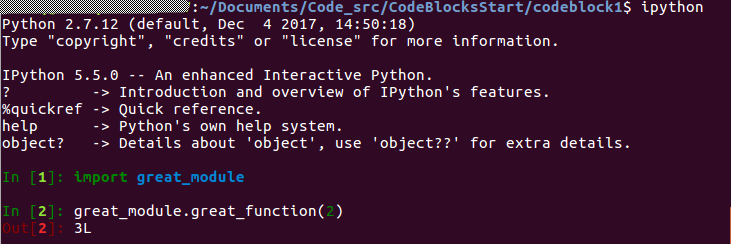# Python调用c++

Python与c++通信，作者描述了Python与c++相互通信的方法，依据文章所述，做了相关的实验，在此转述作者文章并记录自己的学习过程。
1. Python调用c++（基础篇）
2. Python调用c++（高级篇，使用swig工具）

# Python调用c++

return a + 1;
}

from great_module import great_function
great_function(2)
3

#include <Python.h>

int great_function(int a) {
return a + 1;
}

static PyObject * _great_function(PyObject *self, PyObject *args)
{
int _a;
int res;

if (!PyArg_ParseTuple(args, "i", &_a))
return NULL;
res = great_function(_a);
return PyLong_FromLong(res);
}

static PyMethodDef GreateModuleMethods[] = {
{
"great_function",
_great_function,
METH_VARARGS,
""
},
{NULL, NULL, 0, NULL}
};

PyMODINIT_FUNC initgreat_module(void) {
(void) Py_InitModule("great_module", GreateModuleMethods);
}

• 包裹函数_great_function。它负责将Python的参数转化为C的参数（PyArg_ParseTuple），调用实际的great_function，并处理great_function的返回值，最终返回给Python环境。
• 第三个参数的含义是参数变长，第四个参数是一个说明性的字符串。导出表总是以{NULL, NULL, 0, NULL}结束。
• 导出函数initgreat_module。这个的名字不是任取的，是你的module名称添加前缀init。导出函数中将模块名称与导出表进行连接。

• 所有Python元素，module、function、tuple、string等等，实际上都是PyObject。C语言里操纵它们，一律使用PyObject *。
• 也可以创建Python类型的变量，使用PyXXX_New可以创建类型为XXX的变量。
• 若a是Tuple，则a[i] = b对应于 PyTuple_SetItem(a,i,b)，有理由相信还有一个函数PyTuple_GetItem完成取得某一项的值。
• 不仅Python语言很优雅，Python的库函数API也非常优雅。

gcc -fPIC -shared great_module.c -o great_module.so -I/usr/include/python2.7/ -lpython2.7# Python调用c++高级（swig）

SWIG的工作方式：
SWIG本质上是个代码生成器，为C/C++程序生成到其他语言的包装代码(wrapper code)，这些包装代码里会利用各语言提供的C API，将C/C++程序中的内容暴露给相应语言。为了生成这些包装代码，SWIG需要一个接口描述文件，描述将什么样的接口暴露给其他语言。SWIG的 接口描述文件可以包含以下内容：1)ANSI C函数原型声明 2)ANSI C变量声明 3) SWIG指示器(directive)相关内容。SWIG可以直接接受”.h”头文件做为接口描述文件。在有了接口描述文件后，就可以利用swig命令生 成包装代码了，然后将包装代码编译链接成可被其他语言调用的库。
SWIG对Python支持到何种程度？

SWIG包含的内容

## 1. c库

//example.h
int fact(int n); 
//example.c
#include "example.h"

int fact(int n) {
if (n < 0){ /* This should probably return an error, but this is simpler */
return 0;
}
if (n == 0) {
return 1;
}
else {
/* testing for overflow would be a good idea here */
return n * fact(n-1);
}
}  

## 1.2. 利用swig对c库进行包装

### 1.2.1 写一个.i文件进行swig模块的定义

swig会运行.i文件来生成Python包的前身，然后使用编译器来将这个前身生成Python的包(.so文件)，就可以import它了。

/* File: example.i */
%module example

%{
#define SWIG_FILE_WITH_INIT
#include "example.h"
%}

int fact(int n);


### 1.2.2 通过命令行来swig来创建python包的前身

c源码的话：

swig -python example.i

### 1.2.3 编译生成python包

#### 1.2.3.1 直接使用Linux下的c++编译器

gcc -fPIC -I/usr/include/python2.7 -shared -o _example.so example_wrap.c example.c

#### 1.2.3.2 使用python.distutils生成模块动态库

#!/usr/bin/env python

"""
setup.py file for SWIG example
"""

from distutils.core import setup, Extension

example_module = Extension('_example',
sources=['example_wrap.c', 'example.c'],
)

setup (name = 'example',
version = '0.1',
author      = "SWIG Docs",
description = """Simple swig example from docs""",
ext_modules = [example_module],
py_modules = ["example"],
)  

%PYTHON_ROOT%\python setup.py build_ext –inplace

python setup.py build_ext –inplace

## 2. c++库

//example.h
int fact(int n); 
//example.cpp
#include "example.h"

int fact(int n) {
if (n < 0){ /* This should probably return an error, but this is simpler */
return 0;
}
if (n == 0) {
return 1;
}
else {
/* testing for overflow would be a good idea here */
return n * fact(n-1);
}
}  

## 2.2. 利用swig对c++库进行包装

### 2.2.1 写一个.i文件进行swig模块的定义

/* File: example.i */
%module example

%{
#define SWIG_FILE_WITH_INIT
#include "example.h"
%}

int fact(int n);


### 2.2.2 通过命令行来swig来创建python包的前身

swig -c++ -python example.i

### 2.2.3 编译生成python包

#### 2.2.3.1 直接使用Linux下的c++编译器

g++ -fPIC -shared example_wrap.cxx example.cpp -o _example.so -I/usr/include/python2.7/ -lpython2.7

#### 2.2.3.2 使用python.distutils生成模块动态库

#!/usr/bin/env python

"""
setup.py file for SWIG example
"""

from distutils.core import setup, Extension

example_module = Extension('_example',
sources=['example_wrap.cxx', 'example.cpp'],
)

setup (name = 'example',
version = '0.1',
author      = "SWIG Docs",
description = """Simple swig example from docs""",
ext_modules = [example_module],
py_modules = ["example"],
)  

%PYTHON_ROOT%\python setup.py build_ext –inplace

python setup.py build_ext –inplace

# 3 python调用生成的_example.so

ipython
Input: import example
Input: example.fact(5)
Output: 120